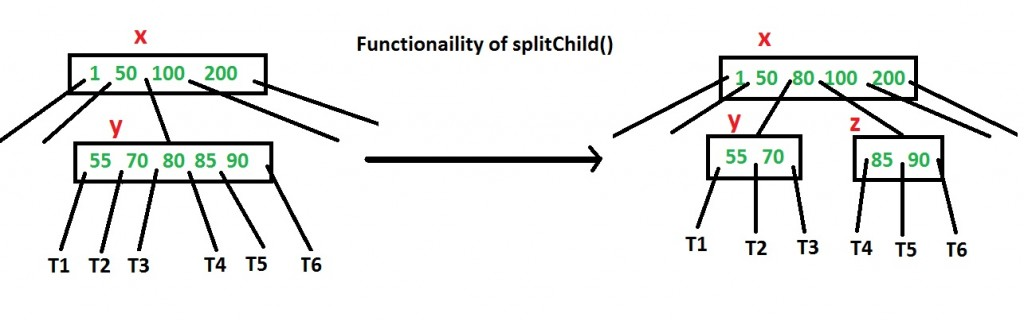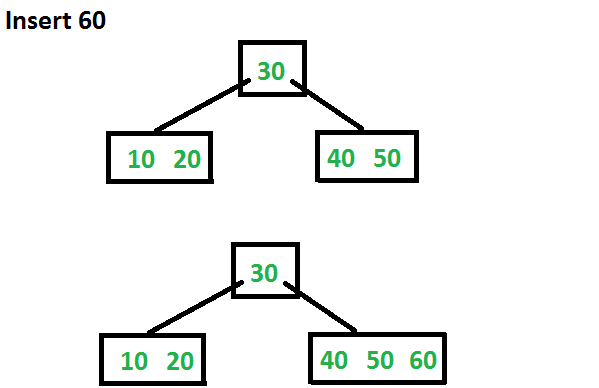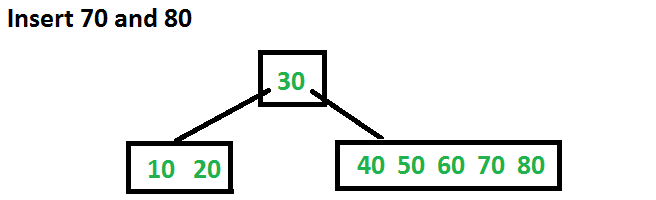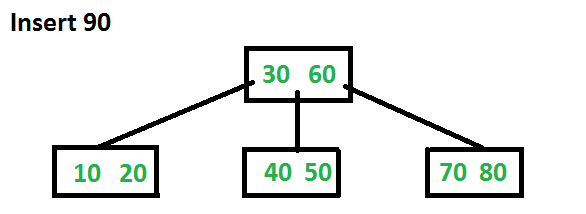# Insert Operation in B-Tree

• Difficulty Level : Hard
• Last Updated : 11 Aug, 2021

In the previous post, we introduced B-Tree. We also discussed search() and traverse() functions.
In this post, insert() operation is discussed. A new key is always inserted at the leaf node. Let the key to be inserted be k. Like BST, we start from the root and traverse down till we reach a leaf node. Once we reach a leaf node, we insert the key in that leaf node. Unlike BSTs, we have a predefined range on the number of keys that a node can contain. So before inserting a key to the node, we make sure that the node has extra space.

How to make sure that a node has space available for a key before the key is inserted? We use an operation called splitChild() that is used to split a child of a node. See the following diagram to understand split. In the following diagram, child y of x is being split into two nodes y and z. Note that the splitChild operation moves a key up and this is the reason B-Trees grow up, unlike BSTs which grow down.As discussed above, to insert a new key, we go down from root to leaf. Before traversing down to a node, we first check if the node is full. If the node is full, we split it to create space. Following is the complete algorithm.

Insertion
1) Initialize x as root.
2) While x is not leaf, do following
..a) Find the child of x that is going to be traversed next. Let the child be y.
..b) If y is not full, change x to point to y.
..c) If y is full, split it and change x to point to one of the two parts of y. If k is smaller than mid key in y, then set x as the first part of y. Else second part of y. When we split y, we move a key from y to its parent x.
3) The loop in step 2 stops when x is leaf. x must have space for 1 extra key as we have been splitting all nodes in advance. So simply insert k to x.

Note that the algorithm follows the Cormen book. It is actually a proactive insertion algorithm where before going down to a node, we split it if it is full. The advantage of splitting before is, we never traverse a node twice. If we don’t split a node before going down to it and split it only if a new key is inserted (reactive), we may end up traversing all nodes again from leaf to root. This happens in cases when all nodes on the path from the root to leaf are full. So when we come to the leaf node, we split it and move a key up. Moving a key up will cause a split in parent node (because the parent was already full). This cascading effect never happens in this proactive insertion algorithm. There is a disadvantage of this proactive insertion though, we may do unnecessary splits.

Let us understand the algorithm with an example tree of minimum degree ‘t’ as 3 and a sequence of integers 10, 20, 30, 40, 50, 60, 70, 80 and 90 in an initially empty B-Tree.
Initially root is NULL. Let us first insert 10.Let us now insert 20, 30, 40 and 50. They all will be inserted in root because the maximum number of keys a node can accommodate is 2*t – 1 which is 5.Let us now insert 60. Since root node is full, it will first split into two, then 60 will be inserted into the appropriate child.Let us now insert 70 and 80. These new keys will be inserted into the appropriate leaf without any split.Let us now insert 90. This insertion will cause a split. The middle key will go up to the parent.Following is C++ implementation of the above proactive algorithm.

## C++

 `// C++ program for B-Tree insertion``#include``using` `namespace` `std;` `// A BTree node``class` `BTreeNode``{``    ``int` `*keys;  ``// An array of keys``    ``int` `t;      ``// Minimum degree (defines the range for number of keys)``    ``BTreeNode **C; ``// An array of child pointers``    ``int` `n;     ``// Current number of keys``    ``bool` `leaf; ``// Is true when node is leaf. Otherwise false``public``:``    ``BTreeNode(``int` `_t, ``bool` `_leaf);   ``// Constructor` `    ``// A utility function to insert a new key in the subtree rooted with``    ``// this node. The assumption is, the node must be non-full when this``    ``// function is called``    ``void` `insertNonFull(``int` `k);` `    ``// A utility function to split the child y of this node. i is index of y in``    ``// child array C[].  The Child y must be full when this function is called``    ``void` `splitChild(``int` `i, BTreeNode *y);` `    ``// A function to traverse all nodes in a subtree rooted with this node``    ``void` `traverse();` `    ``// A function to search a key in the subtree rooted with this node.``    ``BTreeNode *search(``int` `k);   ``// returns NULL if k is not present.` `// Make BTree friend of this so that we can access private members of this``// class in BTree functions``friend` `class` `BTree;``};` `// A BTree``class` `BTree``{``    ``BTreeNode *root; ``// Pointer to root node``    ``int` `t;  ``// Minimum degree``public``:``    ``// Constructor (Initializes tree as empty)``    ``BTree(``int` `_t)``    ``{  root = NULL;  t = _t; }` `    ``// function to traverse the tree``    ``void` `traverse()``    ``{  ``if` `(root != NULL) root->traverse(); }` `    ``// function to search a key in this tree``    ``BTreeNode* search(``int` `k)``    ``{  ``return` `(root == NULL)? NULL : root->search(k); }` `    ``// The main function that inserts a new key in this B-Tree``    ``void` `insert(``int` `k);``};` `// Constructor for BTreeNode class``BTreeNode::BTreeNode(``int` `t1, ``bool` `leaf1)``{``    ``// Copy the given minimum degree and leaf property``    ``t = t1;``    ``leaf = leaf1;` `    ``// Allocate memory for maximum number of possible keys``    ``// and child pointers``    ``keys = ``new` `int``[2*t-1];``    ``C = ``new` `BTreeNode *[2*t];` `    ``// Initialize the number of keys as 0``    ``n = 0;``}` `// Function to traverse all nodes in a subtree rooted with this node``void` `BTreeNode::traverse()``{``    ``// There are n keys and n+1 children, traverse through n keys``    ``// and first n children``    ``int` `i;``    ``for` `(i = 0; i < n; i++)``    ``{``        ``// If this is not leaf, then before printing key[i],``        ``// traverse the subtree rooted with child C[i].``        ``if` `(leaf == ``false``)``            ``C[i]->traverse();``        ``cout << ``" "` `<< keys[i];``    ``}` `    ``// Print the subtree rooted with last child``    ``if` `(leaf == ``false``)``        ``C[i]->traverse();``}` `// Function to search key k in subtree rooted with this node``BTreeNode *BTreeNode::search(``int` `k)``{``    ``// Find the first key greater than or equal to k``    ``int` `i = 0;``    ``while` `(i < n && k > keys[i])``        ``i++;` `    ``// If the found key is equal to k, return this node``    ``if` `(keys[i] == k)``        ``return` `this``;` `    ``// If key is not found here and this is a leaf node``    ``if` `(leaf == ``true``)``        ``return` `NULL;` `    ``// Go to the appropriate child``    ``return` `C[i]->search(k);``}` `// The main function that inserts a new key in this B-Tree``void` `BTree::insert(``int` `k)``{``    ``// If tree is empty``    ``if` `(root == NULL)``    ``{``        ``// Allocate memory for root``        ``root = ``new` `BTreeNode(t, ``true``);``        ``root->keys = k;  ``// Insert key``        ``root->n = 1;  ``// Update number of keys in root``    ``}``    ``else` `// If tree is not empty``    ``{``        ``// If root is full, then tree grows in height``        ``if` `(root->n == 2*t-1)``        ``{``            ``// Allocate memory for new root``            ``BTreeNode *s = ``new` `BTreeNode(t, ``false``);` `            ``// Make old root as child of new root``            ``s->C = root;` `            ``// Split the old root and move 1 key to the new root``            ``s->splitChild(0, root);` `            ``// New root has two children now.  Decide which of the``            ``// two children is going to have new key``            ``int` `i = 0;``            ``if` `(s->keys < k)``                ``i++;``            ``s->C[i]->insertNonFull(k);` `            ``// Change root``            ``root = s;``        ``}``        ``else`  `// If root is not full, call insertNonFull for root``            ``root->insertNonFull(k);``    ``}``}` `// A utility function to insert a new key in this node``// The assumption is, the node must be non-full when this``// function is called``void` `BTreeNode::insertNonFull(``int` `k)``{``    ``// Initialize index as index of rightmost element``    ``int` `i = n-1;` `    ``// If this is a leaf node``    ``if` `(leaf == ``true``)``    ``{``        ``// The following loop does two things``        ``// a) Finds the location of new key to be inserted``        ``// b) Moves all greater keys to one place ahead``        ``while` `(i >= 0 && keys[i] > k)``        ``{``            ``keys[i+1] = keys[i];``            ``i--;``        ``}` `        ``// Insert the new key at found location``        ``keys[i+1] = k;``        ``n = n+1;``    ``}``    ``else` `// If this node is not leaf``    ``{``        ``// Find the child which is going to have the new key``        ``while` `(i >= 0 && keys[i] > k)``            ``i--;` `        ``// See if the found child is full``        ``if` `(C[i+1]->n == 2*t-1)``        ``{``            ``// If the child is full, then split it``            ``splitChild(i+1, C[i+1]);` `            ``// After split, the middle key of C[i] goes up and``            ``// C[i] is splitted into two.  See which of the two``            ``// is going to have the new key``            ``if` `(keys[i+1] < k)``                ``i++;``        ``}``        ``C[i+1]->insertNonFull(k);``    ``}``}` `// A utility function to split the child y of this node``// Note that y must be full when this function is called``void` `BTreeNode::splitChild(``int` `i, BTreeNode *y)``{``    ``// Create a new node which is going to store (t-1) keys``    ``// of y``    ``BTreeNode *z = ``new` `BTreeNode(y->t, y->leaf);``    ``z->n = t - 1;` `    ``// Copy the last (t-1) keys of y to z``    ``for` `(``int` `j = 0; j < t-1; j++)``        ``z->keys[j] = y->keys[j+t];` `    ``// Copy the last t children of y to z``    ``if` `(y->leaf == ``false``)``    ``{``        ``for` `(``int` `j = 0; j < t; j++)``            ``z->C[j] = y->C[j+t];``    ``}` `    ``// Reduce the number of keys in y``    ``y->n = t - 1;` `    ``// Since this node is going to have a new child,``    ``// create space of new child``    ``for` `(``int` `j = n; j >= i+1; j--)``        ``C[j+1] = C[j];` `    ``// Link the new child to this node``    ``C[i+1] = z;` `    ``// A key of y will move to this node. Find the location of``    ``// new key and move all greater keys one space ahead``    ``for` `(``int` `j = n-1; j >= i; j--)``        ``keys[j+1] = keys[j];` `    ``// Copy the middle key of y to this node``    ``keys[i] = y->keys[t-1];` `    ``// Increment count of keys in this node``    ``n = n + 1;``}` `// Driver program to test above functions``int` `main()``{``    ``BTree t(3); ``// A B-Tree with minimum degree 3``    ``t.insert(10);``    ``t.insert(20);``    ``t.insert(5);``    ``t.insert(6);``    ``t.insert(12);``    ``t.insert(30);``    ``t.insert(7);``    ``t.insert(17);` `    ``cout << ``"Traversal of the constructed tree is "``;``    ``t.traverse();` `    ``int` `k = 6;``    ``(t.search(k) != NULL)? cout << ``"\nPresent"` `: cout << ``"\nNot Present"``;` `    ``k = 15;``    ``(t.search(k) != NULL)? cout << ``"\nPresent"` `: cout << ``"\nNot Present"``;` `    ``return` `0;``}`

Output:

```Traversal of the constructed tree is  5 6 7 10 12 17 20 30
Present
Not Present```

References:
Introduction to Algorithms 3rd Edition by Clifford Stein, Thomas H. Cormen, Charles E. Leiserson, Ronald L. Rivest
http://www.cs.utexas.edu/users/djimenez/utsa/cs3343/lecture17.html